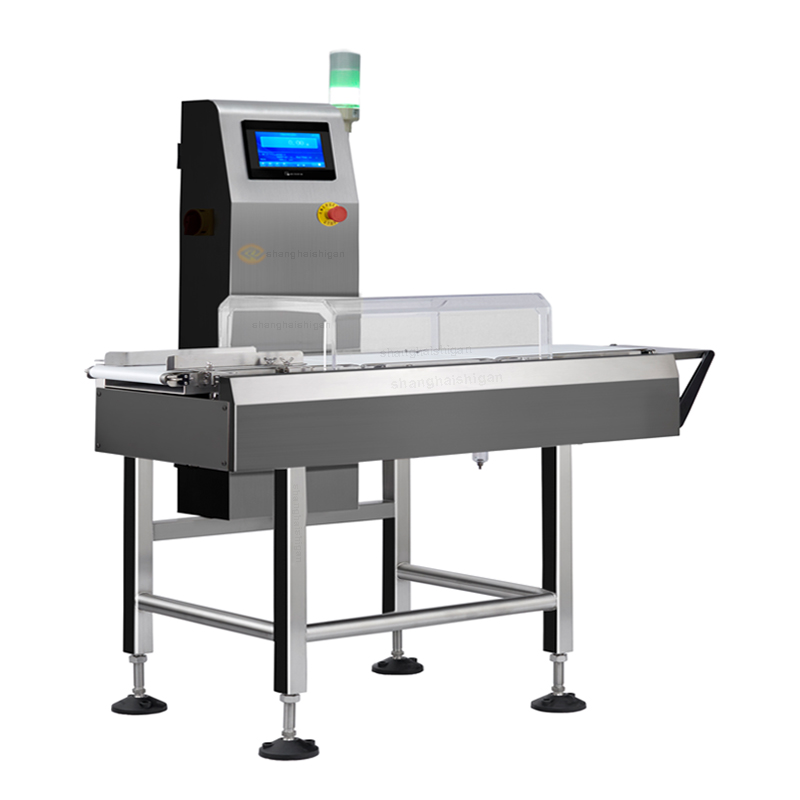# Two precision test methods for check weighing scale

2020-07-18 15:45:27

Check weighing scale(also called online checkweigher,weighing scale and sorting machine) is a key equipment, automatic production line is very it has high precision, high speed, can save a single product weight and total weight per batch products, and other functions, but in the use of inspection in the process of weighing scales, want to ensure that every batch of products can achieve accurate measurement, it needs to check to test the accuracy of weighing scales, and precise test that how to correct?The following small series of Shanghai practical for you to collect two test methods, I hope to help you.The first test is to pass the same product 10 or 20 times, making sure that each pass is in the same direction as you would in production.

1. Record each data. Some check scales can automatically record and calculate standard deviation.If the balance cannot be calculated automatically, it can be calculated using the STDEV formula in Excel.

2. After the standard deviation, multiply by 6 to obtain the unconfidence interval (a calculation method for the uncertainty of dynamic weight detection globally accepted).

3. Retrieve the TNE(-T1) in the table below. For example, if your product weighs 200 grams, the TNE(-T1) is 5% or 9g.

4. Now calculate a quarter of this 9g, which is 0.25 x 9g = 2.25g.This is your limit for repeatability.

5. If your initial unconfidence interval is greater than 2.25g, then the weigher is not available under this setting.

This test is required for all different types and weights of products to be checked on the weigher.To do this better, repeat the test every once in a while, recording and analyzing trends for each test to see how the results change over time.Based on this trend, you can determine how often you need to run the test.These records, along with trend analysis, are also valid evidence of the property risk assessment of the weigher users.

The second test is also carried out using the product under test. In addition, a calibrated static balance is also needed.The resolution of the static balance should be the same as that of the check weigher, or higher.This inspection is required before, after, and at the end of a batch.Again, this test can do trend analysis for further risk assessment.

1. Use correction weights of similar weight to the product to check whether the static scale can be weighed correctly.

2. Pass the running check weigher, and then put it on the static scale for weighing.Compare the weight value on the checking scale with that on the static scale.If the difference is greater than 1/5 of the TNE, this check weigher is not applicable.When the batch is in progress, some checkweighers have a built-in weight check function, which removes the product for testing only and displays the weight on the screen at a constant rate for testing purposes.

3. Repeat this test at least once to check the repeatability of the weigher.

For example, if our product has a rated weight of 200g, the approved weight on the weighing scale is 200.4 g, the same product is put on the static scale and the weighing shows 199.7 g.The difference is 0.7 grams.We know that the TNE of a 200 g product is 9 g.One in five is 1.8 grams.When 0.7g is less than 1.8 g, the check weigher can be accurately measured and used normally.

For each batch of product testing to be accurate, you need to run these two tests and write them into your standard workflow.Repeat the two tests after a certain amount of time, and once you pass the tests, you can safely use the weigher.

Shanghai Shigan is a professional check weighing scale manufactures.Hope these two methods can bring you help, if you still want to understand the content, welcome to click here to consult!

Related Searches